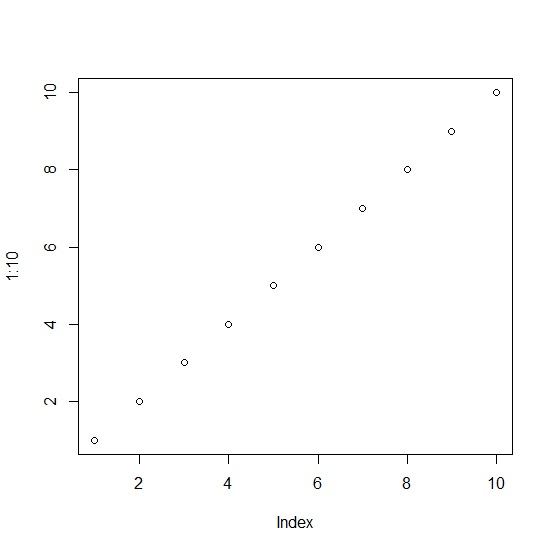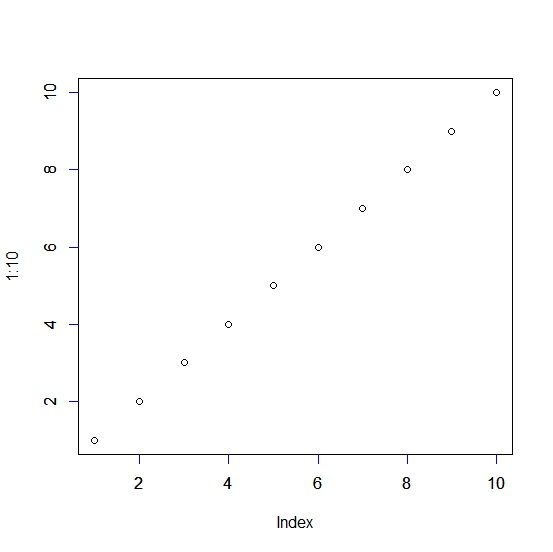# How to change the axis ticks color in base R plot?

To change the axis ticks color in base R plot, we can use axis function after creating the plot. For example if we want to change the color of X-axis to red then we can use the command given below −

axis(1,col.ticks="red")

If we want to change the color of Y-axis to red then we can use the command given below −

axis(2,col.ticks="red")


Check out the below given example to understand how it works.

## Example

To change the axis ticks color in base R plot, use the code given below −

plot(1:10)

## Output

If you execute the above given code, it generates the following output −To change the axis ticks color in base R plot, add the following code to the above snippet −

plot(1:10)
axis(1,col.ticks="blue")
axis(2,col.ticks="blue")

## Output

If you execute all the above given codes as a single program, it generates the following output −